Next: 8.5 Mapping Up: 8. Data Processing Level: Previous: 8.3 Common Processing Steps

Subsections

# 8.4 Photometry

The conversion from power on the detector in units of W to flux density in Jy or surface brightness in MJysr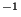is presented. The processing of measurements of PHT-P and PHT-C on one hand and PHT-S on the other hand have been separated due to the distinct photometric calibration schemes. Finally, the writing of the resulting data to product files is briefly described.

## 8.4.1 Determine source in-band power for chopped observations

Operation:  Extract the source power from the powers of the different chopper positions.

The in-band powers of on-source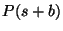with uncertainty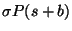and off-source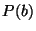with uncertainty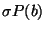are read from the SPD product. For each measurement the source power is computed: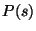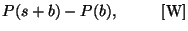(8.3)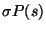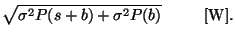(8.4)

## 8.4.2 Summation of the in-band powers over all PHT-C detector pixels

Operation:  Derive the sum of the in-band powers of all pixels for a given PHT-C array.

In case the observer requested the measurement of a point source flux with the PHT-C detector arrays, the total [source+background] power as well as the background power on the array is determined by summing the respective powers over all pixels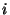.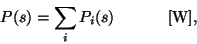(8.5)

and for the [source+background] and background power: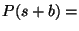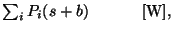(8.6)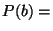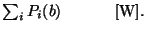(8.7)

The uncertainties are computed according to: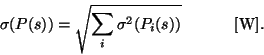(8.8)

The relations for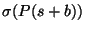and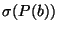are similar.

## 8.4.3 Fit two-dimensional Gaussian to point sources in the C-arrays

Operation: In case the observer has requested a point source measurement, a 2 dimensional Gaussian function is fitted to the intensity pattern on the array. This processing is done in addition to the sum of all pixel in-band powers (Section 8.4.2).

Caveat: This method of providing point source photometry is not scientifically validated. In particular in the case of faint sources and noisy data, the derived fluxes and uncertainties are not reliable.

To secure a converging fit, an interpolation is performed whenever there are undefined in-band powers for some pixels. The fitting of the 2 dimensional Gaussian itself is performed using standard iterative fitting routines provided by the NAG mathematical routines library (routines E04FDF and E04YCF).

The following parameters are obtained:

•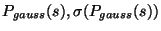: peak power value and associated error (indicated with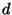in Section 8.4.5);
•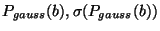: background power value and error (indicated with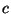in Section 8.4.5);
•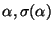: x offset of peak in arcsec and error;
•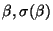: y offset of peak in arcsec and error;
•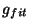: goodness of fit
• fit status, if status=0, fit is successful.

Details of the procedure are given in the next sections (Sections 8.4.4 and 8.4.5).

## 8.4.4 Interpolate missing pixels

The fitting routine described in Section 8.4.5 requires only valid pixel intensities on the detector array. Interpolation is necessary in case there are bad' data pixels.

A check is performed to determine whether there is a sufficient number of good pixels for interpolation. For C200 one pixel is allowed to be missing. For C100 the criteria are (1) the presence of the centre pixel (pixel 5) where the source is expected to be and (2) there must be at least 2 good pixels on any side of the array. Criterion (2) is imposed to avoid interpolation using an already interpolated value.

The rules for interpolation are


C200:  a b    :   a = b + c - d
c d

C100:  a b c  :   b = (a + c)/2
d e f
g h i      a = b + d - (c + g)/2


where the individual pixels are designated by letters. Note that there is a rotational symmetry about each side; only one orientation is given. The accuracy of the method depends on

• the location of the source in the array,
• the presence of any extended features, and
• the uniformity of the pixel response.

## 8.4.5 Determination of Gaussian parameters

The height of the source peak, its position, and the background level is obtained by fitting a Gaussian function to the data. The accuracy of this method depends on the correctness of the assumption of a Gaussian on top of a constant background. The in-band power distribution is considered as a 2 dimensional array: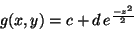where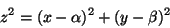and

•is a constant background.
•is the (background subtracted) height of the source peak.
•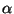is the x offset of the peak from the array centre.
•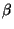is the y offset of the peak from the array centre.

The x and y axes are the first (along spacecraft z-axis) and second (along spacecraft y-axis) dimension of the pixel array, respectively, with origin at the centre of the array. A chi-squared goodness of fit' function is defined as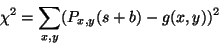The best fit can be found by determining the minimum of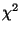.

For C100, estimates of the uncertainties on the parameters can be derived from the Jacobian of the function at the solution. Detailed discussion of the method is beyond the scope of this document; the NAG algorithm E04YCF is used. The nominal uncertainty of the fit is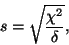where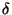is the number of degrees of freedom which is determined by the number of pixels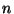, the number of parameters (4), and the number of interpolations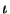performed on C100 (Section 8.4.4):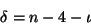Since the position of the peak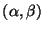is not related to its size or the level of the background on which it is located,and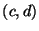are largely independent of each other. Thus adding 2 to the degrees of freedom is justified. This argument implies that the uncertainties for C100 may be overestimated. For C200 it is assumed that: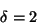The variances are calculated from: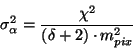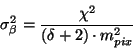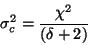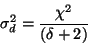where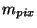is the mean pixel value used to scale into the correct units: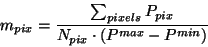The uncertainty of the fit is estimated as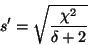### Ancillary data needed

None. See Chapter E04 of the NAG manual.

## 8.4.6 Photometry with PHT-P and PHT-C

Operation:  Convert the mean in-band power on a PHT-P or PHT-C detector (pixel) to a monochromatic flux density (Jy) assuming a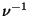or - equivalently - constant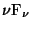spectral energy distribution.

The monochromatic flux density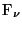in Jy for PHT-P or PHT-C is derived as follows (see Equation 5.10):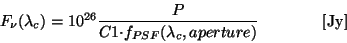(8.9)

with uncertainty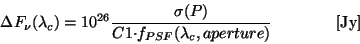(8.10)

where,

•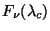in Jy is the flux density at the reference wavelength of the filter, and
•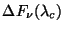in Jy the corresponding uncertainty;
•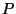in W is the in-band power on the detector in the selected filter band, and
•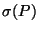in W the corresponding uncertainty;
•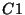in m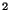Hz is a constant related to each filter band and describes the total transmission of the bandpass along the optical path onto the detector; this includes the size of the mirror, reflections, filter transmission, spectral response of the detector;
•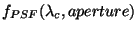is the fraction of the telescope point-spread function falling into a given aperture for PHT-P or onto the full array for PHT-C.

For chopped measurements, the powers,, andare converted to flux densities in Jy. For chopped measurements with C100 only pixel 5 is used for the determination of the source flux. This is different in case of staring mode where the sum over the 9 C100 pixels is employed.

### Ancillary data needed

• power calibration factors C1 for all filters read from Cal-G file PFLUXCONV, see Sections 5.2.5 and 14.20.1.
• reference wavelengths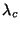for all PHT-P and C bands also stored in PFLUXCONV, see Section 14.20.1.
• point spread function factors are stored in Cal-G file PPPSF for PHT-P and PCPSF f or PHT-C, see Section 14.18. Note: the PCPSF file contains correction factors for the entire array (for staring mode) and for a single pixel (for chopped mode).

## 8.4.7 Surface brightness determination for PHT-P and PHT-C

Operation:  Convert flux density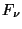in Jy to surface brightness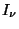in MJysr.

The surface brightness calculation assumes that the point source flux density has been derived. Based on the point source flux density the surface brightness is determined from: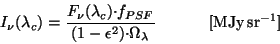(8.11)

with the uncertainty computed according to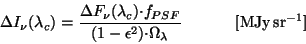(8.12)

where with the same definitions as in the previous sections,

•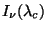in MJysris the surface brightness at the reference wavelength of the filter, and
•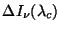in MJysrthe corresponding uncertainty;
•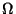in sr is the effective solid angle on the sky of the detector pixel or selected aperture;
• (1-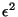)=0.91 (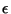=0.30) is the correction for the obscuration by the secondary mirror.

The values of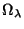were computed by using a model which takes into account the ISO telescope mirrors as well as the physical sizes of the apertures in case of PHT-P or detectors in case of PHT-C. The model provided the 2-dimensional beam profile (or `footprint') of each possible aperture/filter (PHT-P) or pixel/filter (PHT-C) combination. The value ofwas eventually obtained from the integral of the footprint.

### Ancillary data needed

• Effective solid anglesfor each filter/aperture and filter/pixel combination. The values are read from Cal-G files PPOMEGA in case of PHT-P, PC1OMEGA in case of PHT-C100 and PC2OMEGA in case of PHT-C200. See also Appendix A.1.2, and Section 14.21.

## 8.4.8 Write PHT-P point source photometry product

Operation: Write a complete PHT-P point source photometry product.

Write the product FITS header followed by the processed data in a binary table with each record containing the data for a single filter or aperture.

Detailed product descriptions can be found in Sections 13.4 and 13.4.2 (product PPAP).

## 8.4.9 Write PHT-P extended source photometry product

Operation: Write a complete PHT-P extended source photometry product.

Write the product FITS header followed by the processed data in a binary table with each record containing the data for a single filter or aperture.

Detailed product descriptions can be found in Sections 13.4 and 13.4.3 (PPAE) for a single pointing product.

## 8.4.10 Write PHT-C point source photometry product

Operation: Write a complete PHT-C point source photometry product.

Write the product FITS header followed by the processed data in a binary table with each record containing the data for a single filter.

Detailed product descriptions can be found in Sections 13.4 and 13.4.4 (PCAP).

## 8.4.11 Write PHT-C extended source photometry product

Operation: Write a complete PHT-C extended source photometry product.

Write the product FITS header followed by the processed data in a binary table with each record containing the data for a single filter.

Detailed product descriptions can be found in Section 13.4 and 13.4.5 (PCAE) for a single pointing product.Next: 8.5 Mapping Up: 8. Data Processing Level: Previous: 8.3 Common Processing Steps
ISO Handbook Volume IV (PHT), Version 2.0.1, SAI/1999-069/Dc